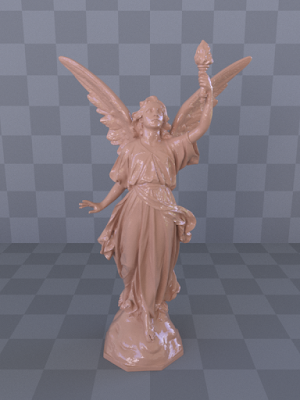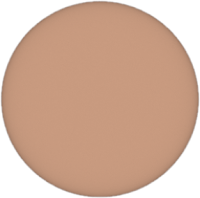### Layered Materials

Most of the materials in real life can be modeled more precisely by using layers of different materials. Some layered surfaces can be analytically solved and converted into a single reflection model. However, vast majority of them cannot be represented this way. Therefore, representing arbitrarily layered materials is quiet challenging. The most obvious way is to simulate all the interactions between layers. Although this is straightforward to implement, it might cause higher variance and also it is not possible to find pdf and bsdf values for $(w_i, w_o)$ pairs, obviously.

Arbitrarily Layered Micro-Facet Surfaces [WW2007] presents a method that unifies arbitrarily layered microfacet surfaces into a single surface model. However, there are some issues with this paper:
• When sampling a direction between layers, a direction is chosen according to the individual brdfs of the surfaces. So, if a layer is rough, the transmitted or reflected direction is chosen accordingly. However, when brdf and pdf is calculated, it is always assumed that the rays are refracted as if the surfaces were smooth (perfect refraction). This is a highly practical approach causing wrong results.
• Term $t$ is not explained at all. So, compensation term is only empirical since the idea behind it is a mystery.
• It is not implementation friendly. Most explanations are vague and it is all up to implementer to work on it.
This paper is more like an influencer for derivation of physically-based models. After reading the paper, I tried to create a simple model which contains two layers:
1. A perfect smooth dielectric surface layer on top.
2. A perfect lambertian surface layer on bottom.
It is a much more simplified setup but a single physically correct brdf is possible for the transmitted light. Let's start to derive:
1. We assume that all the interaction between light and surface happens in the same differential area $d_A$ so that light enters and exit the same point. (Also, one of the assumptions made in the paper.)
2. We want to compute
$L_o = \int_{\Omega}^{} f_{tr} L_i cos(\theta_i) dw_i$
If we differentiate above with respect to $dw_i$, we have
$$ $\frac{dL_o}{dw_i} = f_{tr} L_i cos(\theta_i)$
3. We can write the rendering equation for the lambertian surface layer as in the second step and have $\frac{dL_{o\_ins}}{dw_{i\_ins}} = f_r L_{i\_ins} cos(\theta_{i\_ins})$. However, this time photons bounce and scatter until they lose all their energy. Each time they bounce and scatter, they contribute to differential radiance $\frac{dL_{o\_ins}}{dw_{i\_ins}}$. Let differential irradiance at first interaction on the bottom layer be $\Phi = L_{i\_ins} cos(\theta_{i\_ins})$.
On the first interaction, the amount of energy that will be scattered to $w_{o\_inside}$ is
$\Phi \frac{k_d}{\pi}$
On the second interaction, the amount of energy that will be scattered is
$L_o = \int_{\Omega}^{} \frac{k_d}{\pi} L_i cos(\theta_{i\_ins}) dw_{i\_ins}$ where $L_i = \Phi \frac{k_d}{\pi} F(cos(\theta_{i\_ins}))$
Let's rearrange the terms such that
$L_o = \Phi \frac{k_d}{\pi} \int_{\Omega}^{} \frac{k_d}{\pi} F(cos(\theta_{i\_ins})) cos(\theta_{i\_ins}) dw_{i\_ins}$
To simplify the equation above, let
$A=k_d \int_{\Omega}^{} \frac{1}{\pi} F(cos(\theta_{i\_ins})) cos(\theta_{i\_ins}) dw_{i\_ins}$ so that $L_o = \Phi \frac{k_d}{\pi} A$
It is now easy to see that we can write $L_o = \Phi \frac{k_d}{\pi} A A$ for the third interaction, $L_o = \Phi \frac{k_d}{\pi} A A A$ for the fourth, $L_o = \Phi \frac{k_d}{\pi} A A A A$ for the fifth and so on.
We finally can write
$$ $\frac{dL_{o\_ins}}{dw_{i\_ins}} = L_{i\_ins} cos(\theta_{i\_ins}) \frac{k_d}{\pi} (1 + A + A^2 + A^3+...) = L_{i\_ins} cos(\theta_{i\_ins}) \frac{k_d}{\pi} \frac{1}{1-A}$
4. For perfect refractive surfaces, we know that
$L_{outgoing} = L_{incoming} (1-F) (\frac{n_o}{n_i})^2$
We should multiply the both sides of the equation  by below so that $\frac{dL_{o\_ins}}{dw_{i\_ins}}$ converts to $\frac{dL_{o}}{dw_{i\_ins}}$.
$(\frac{n_{outside}}{n_{dielectric}})^2 (1-F_{out})$
Thus, we have
$$ $\frac{dL_{o}}{dw_{i\_ins}} = (\frac{n_{outside}}{n_{dielectric}})^2 (1-F_{out}) L_{i\_ins} cos(\theta_{i\_ins}) \frac{k_d}{\pi} \frac{1}{1-A}$
5. When light first enters the dielectric
$L_{i\_ins} = L_i (1-F_{in}) (\frac{n_{dielectric}}{n_{outside}})^2$
Plugging equation above into equation , we have
$$ $\frac{dL_{o}}{dw_{i\_ins}}=L_i (1-F_{out}) (1-F_{in}) cos(\theta_{i\_ins}) \frac{k_d}{\pi} \frac{1}{1-A}$
6. Multiply both sides of equation  by
$|\frac{dw_{i\_ins}}{dw_i}|$
We have
$$ $\frac{dL_o}{dw_i}= \frac{dL_o}{dw_{i\_ins}} |\frac{dw_{i\_ins}}{dw_i}|= |\frac{dw_{i\_ins}}{dw_i}| L_i (1-F_{out}) (1-F_{in}) cos(\theta_{i\_ins}) \frac{k_d}{\pi} \frac{1}{1-A}$.
7. Combining equations  and , we have
$$ $f_{tr} = |\frac{dw_{i\_ins}}{dw_i}| (1-F_{out}) (1-F_{in}) cos(\theta_{i\_ins}) \frac{k_d}{\pi} \frac{1}{1-A} \frac{1}{cos(\theta_i)}$
8. We can compute the jacobian geometrically as explained in Microfacet Models for Refraction through Rough Surfaces[WMLT07] and find
$|\frac{dw_{i\_ins}}{dw_i}| = (\frac{n_{outside}}{n_{dielectric}})^2 \frac{cos(\theta_i)}{cos(\theta_{i\_ins})}$
Plugging jacobian into , we have final equation for our transmission brdf
$f_{tr} = (\frac{n_{outside}}{n_{dielectric}})^2 (1-F_{out}) (1-F_{in}) \frac{k_d}{\pi} \frac{1}{1-A}$
For importance sampling, a trivial choice would be to sample the direction in the bottom layer with cosine-weighted sampling and refract it to have $w_o$. However, some samples might be wasted if the sampled directions causes a total internal reflection. To avoid this, $p(w_{i\_ins})=\frac{cos(\theta_{i\_ins})}{\pi sin^2(\theta_{critical})}$ can be used. Also, transformation  $p(w_{i\_ins}) |\frac{dw_{i\_ins}}{dw_i}|=p(w_i)$ should be applied when using this pdf.

However, choosing a direction inside for $\theta \in [0, \theta_{critical}]$ is equivalent of choosing a direction outside for $\theta \in [0, \pi / 2]$. This can be shown as follows.
$p(w_i)=p(w_{i\_ins}) |\frac{dw_{i\_ins}}{dw_i}|=\frac{cos(\theta_{i\_ins})}{\pi sin^2(\theta_{critical})} (\frac{n_{outside}}{n_{dielectric}})^2 \frac{cos(\theta_i)}{cos(\theta_{i\_ins})}$
Since  $sin^2(\theta_{critical})=(\frac{n_{outside}}{n_{dielectric}})^2$, equation above can be simplified to
$p(w_i) = \frac{cos(\theta_i)}{\pi}$
Obviously, using $p(w_{i\_ins})$ inside is equivalent of using cosine-weighted sampling outside. Latter avoids extra refraction calculations.

Glue does the followings for this material:
• It uses monte carlo technique to precompute the integral:
$A=k_d \int_{\Omega}^{} \frac{1}{\pi} F(cos(\theta_{i\_ins})) cos(\theta_{i\_ins}) dw_{i\_ins}$
• Uses cosine-weighted sampling to directly sample $w_o$.
• At every interaction, either reflection or transmission brdf is selected based on fresnel value of $w_i$. While reflection brdf has delta distribution and causes no direct lighting calculations, transmission brdf does the opposite. So, separating them is more efficient.IOR: 1.33IOR: 1.50

Here are some results under constant background light. They are compared with results of full monte carlo simulation and found no difference. The effect of compensation term $A$ becomes more obvious as IOR increases.IOR: 1.0 (Same as lambertian surface)IOR: 1.3 with A=0 (energy loss allowed)IOR: 1.3IOR: 1.5IOR: 1.5 with A=0 (energy loss allowed)IOR: 2.0IOR: 2.0 with A=0 (energy loss allowed)

Relevant implementation is: